# 干货：一文弄懂时间序列预测的基本原理

Quantitative-Finance Time-Series

「Python数据之道」导语：本文内容较长，较为详细的阐述了进行时间序列预测的步骤，有些内容可能暂时用不到或者看不懂，但不要紧，知道有这么一个概念，后续碰到的时候，继续深入学习以及使用就可以。

# 干货 : 一文弄懂时间序列预测的基本原理Photo by Adrian Schwarz on Unsplash

https://nbviewer.jupyter.org/github/leandrovrabelo/tsmodels/blob/master/notebooks/english/Basic Principles for Time Series Forecasting.ipynb

## 平稳序列

• 常数均值
• 常数方差
• 自相关

### 常数均值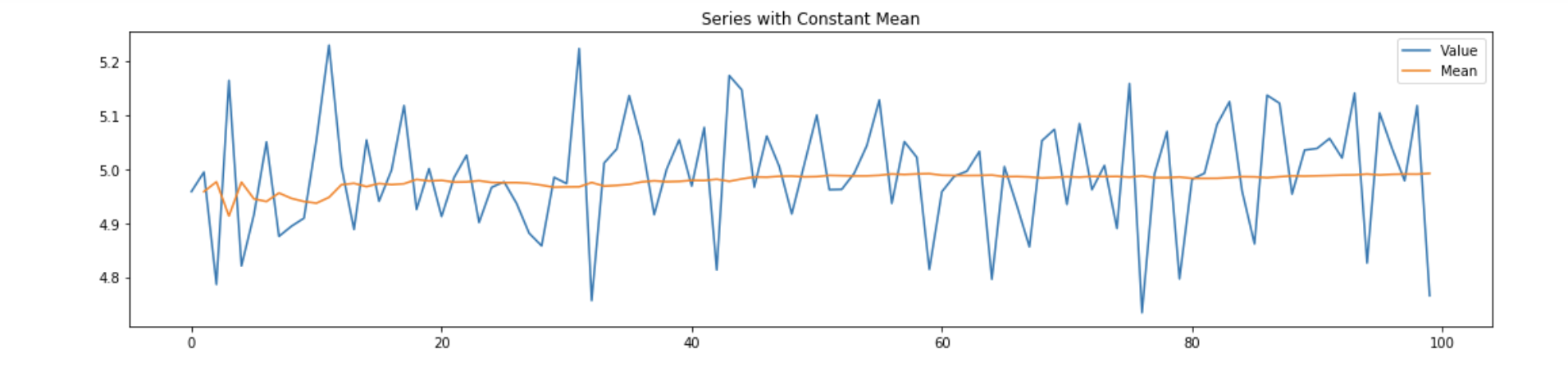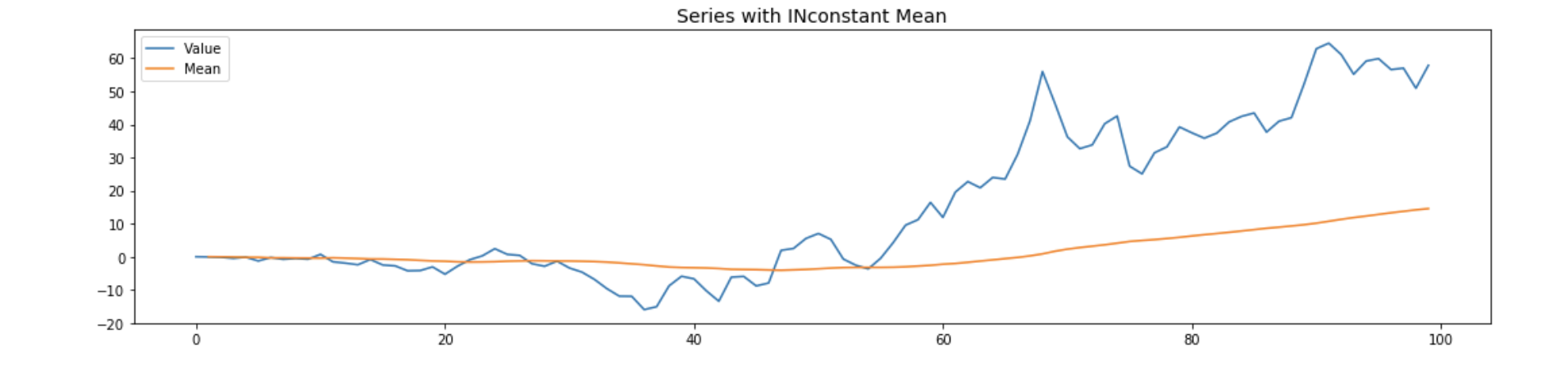### 常数方差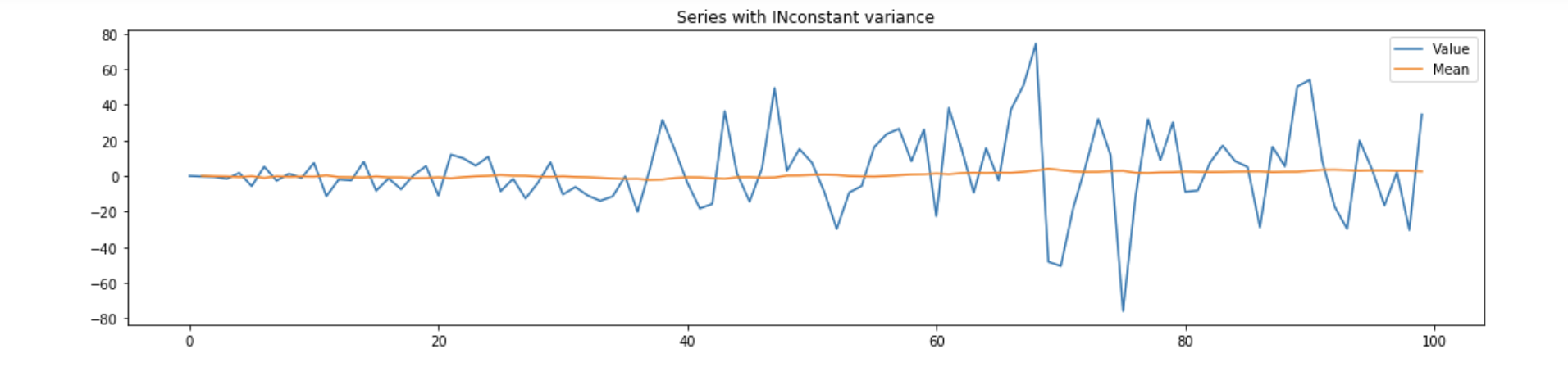### 自相关序列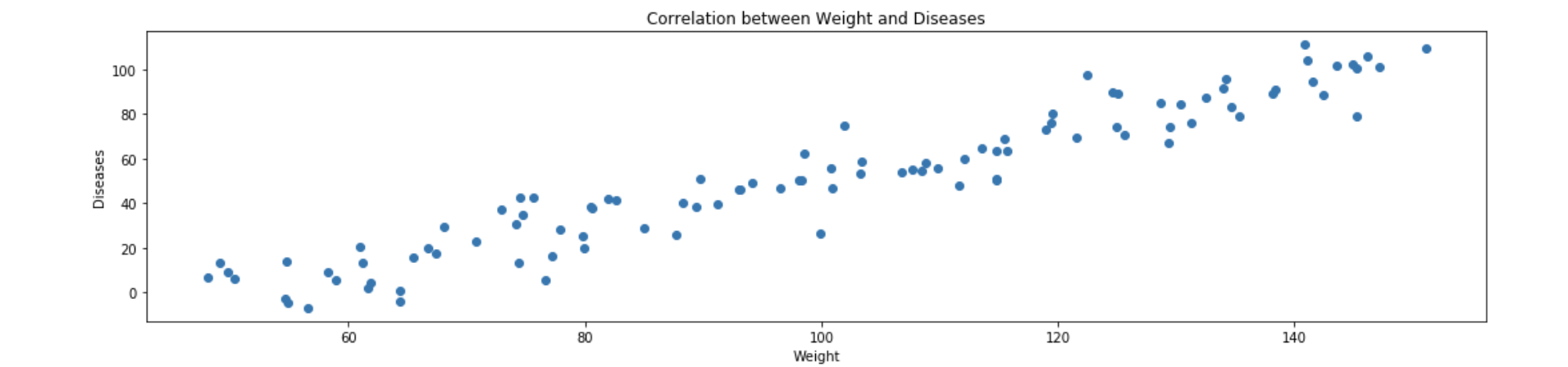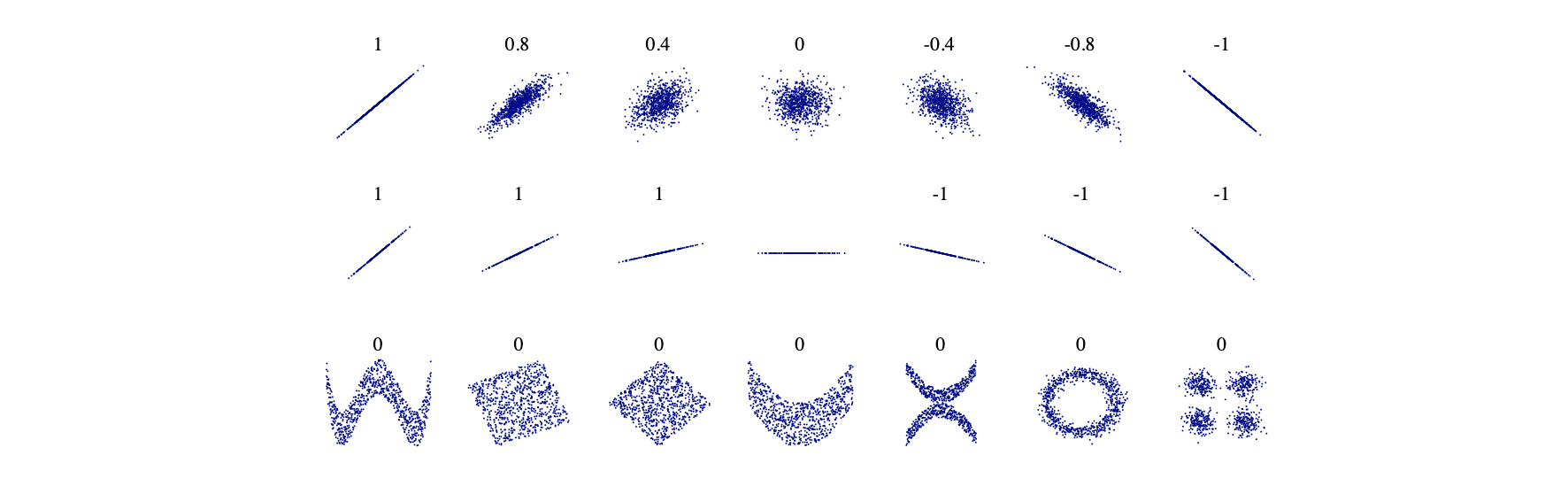## 划分训练集和测试集数据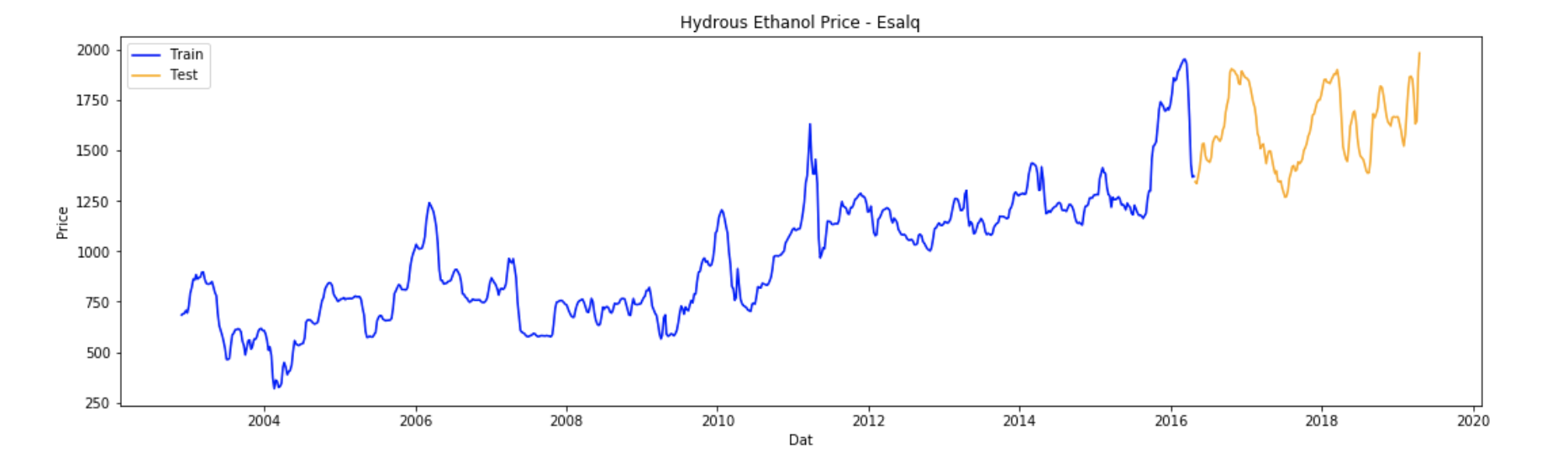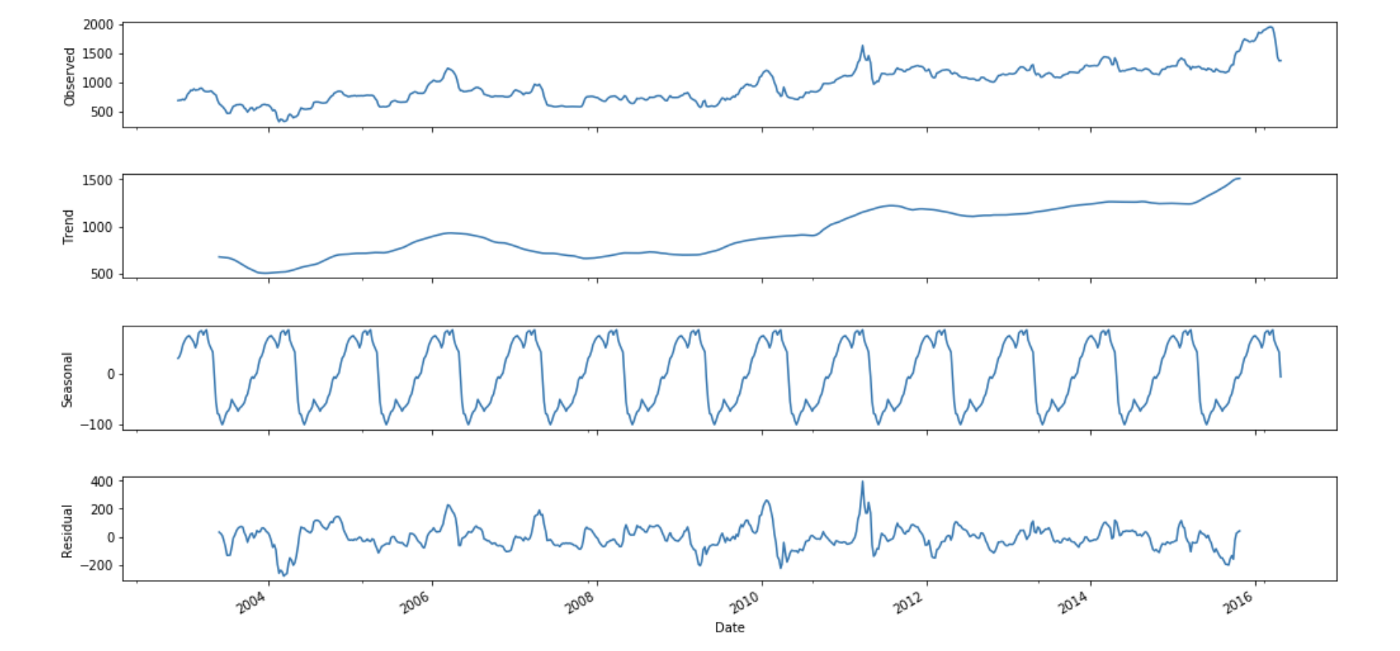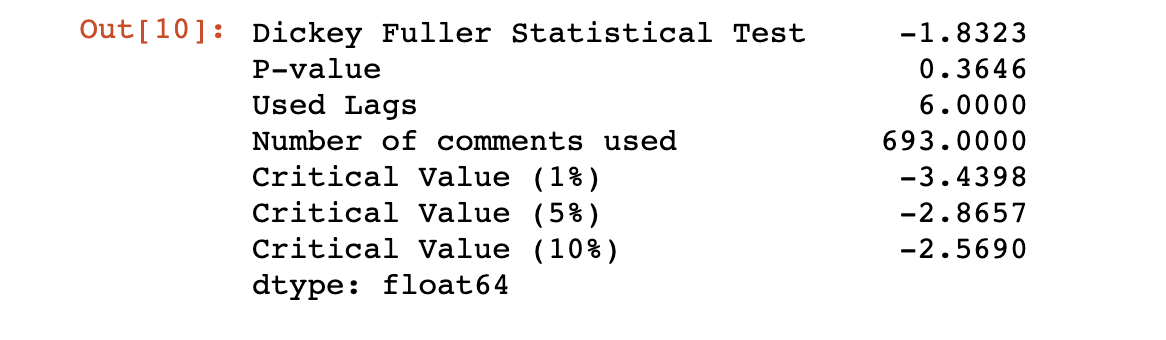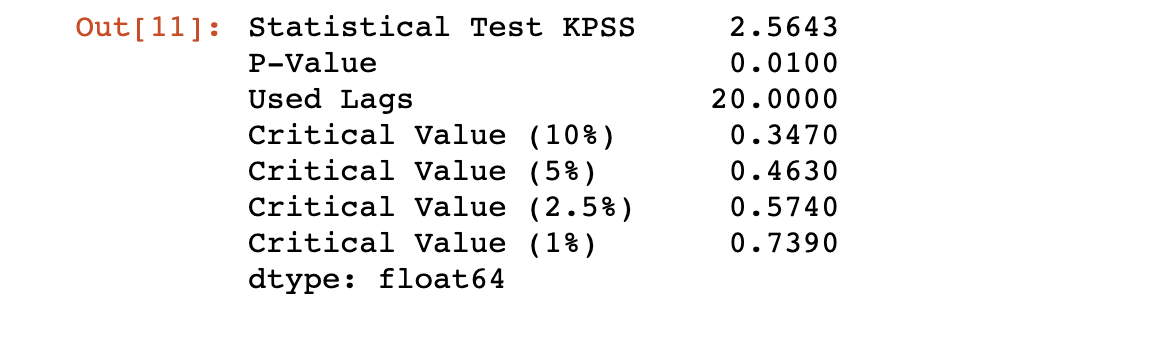KPSS 检验证实了 Dickey-Fuller 检验的正确性，同时也表明该序列不是平稳的，因为 P 值为 1%，统计检验高于任何临界值。

## 将序列转换为平稳状态

### 差分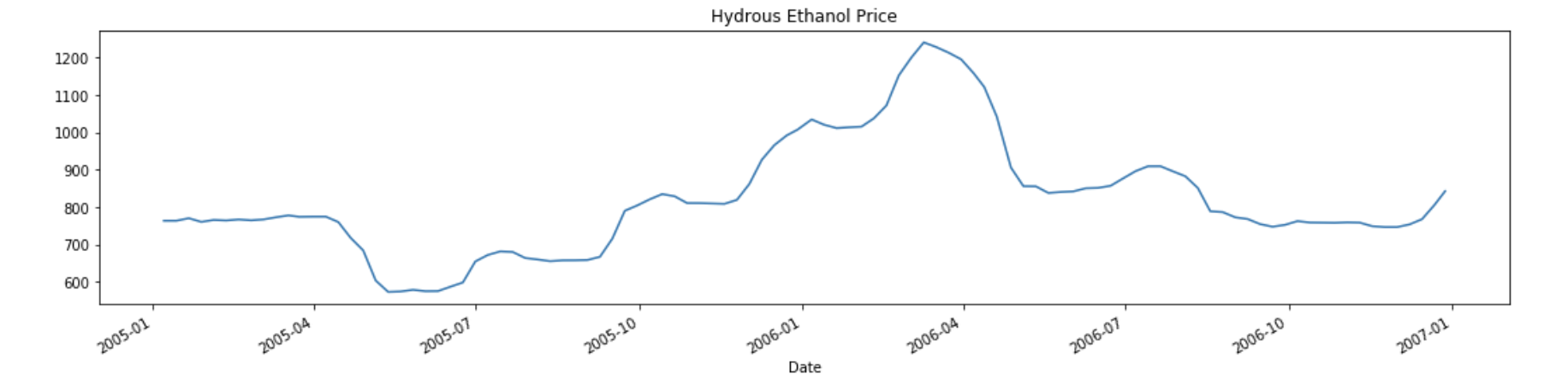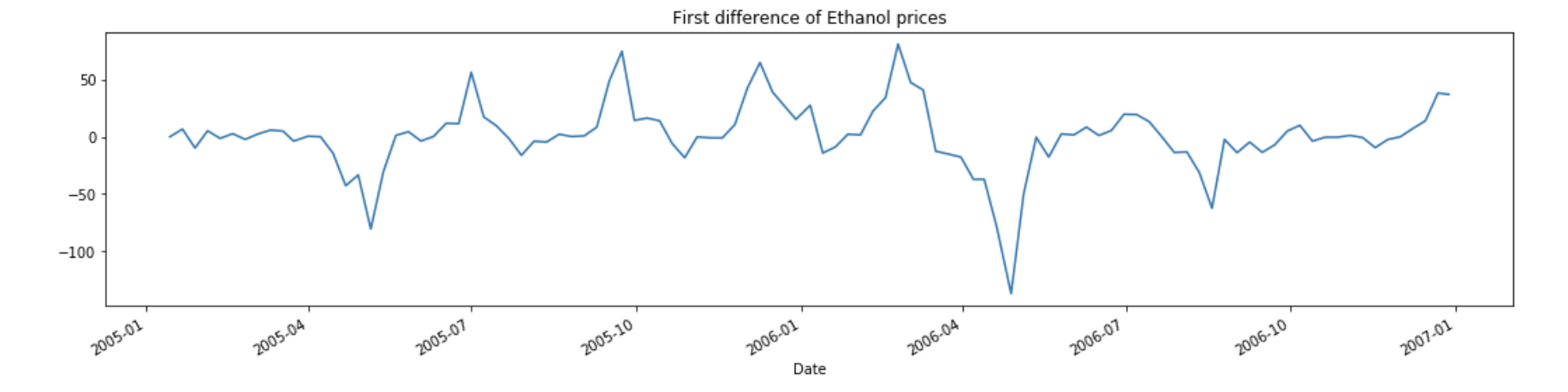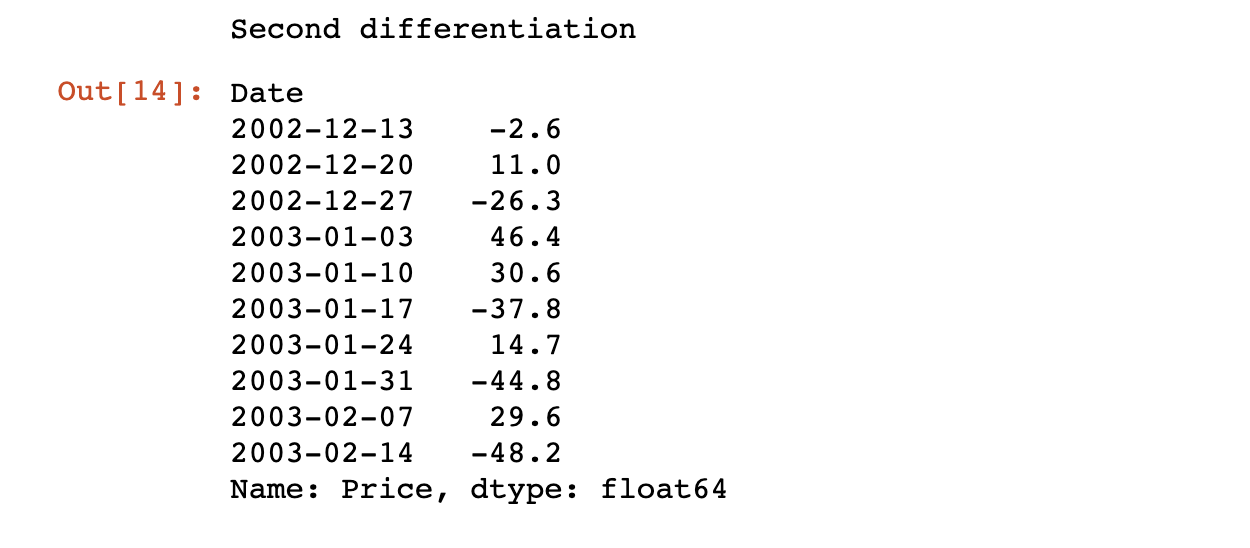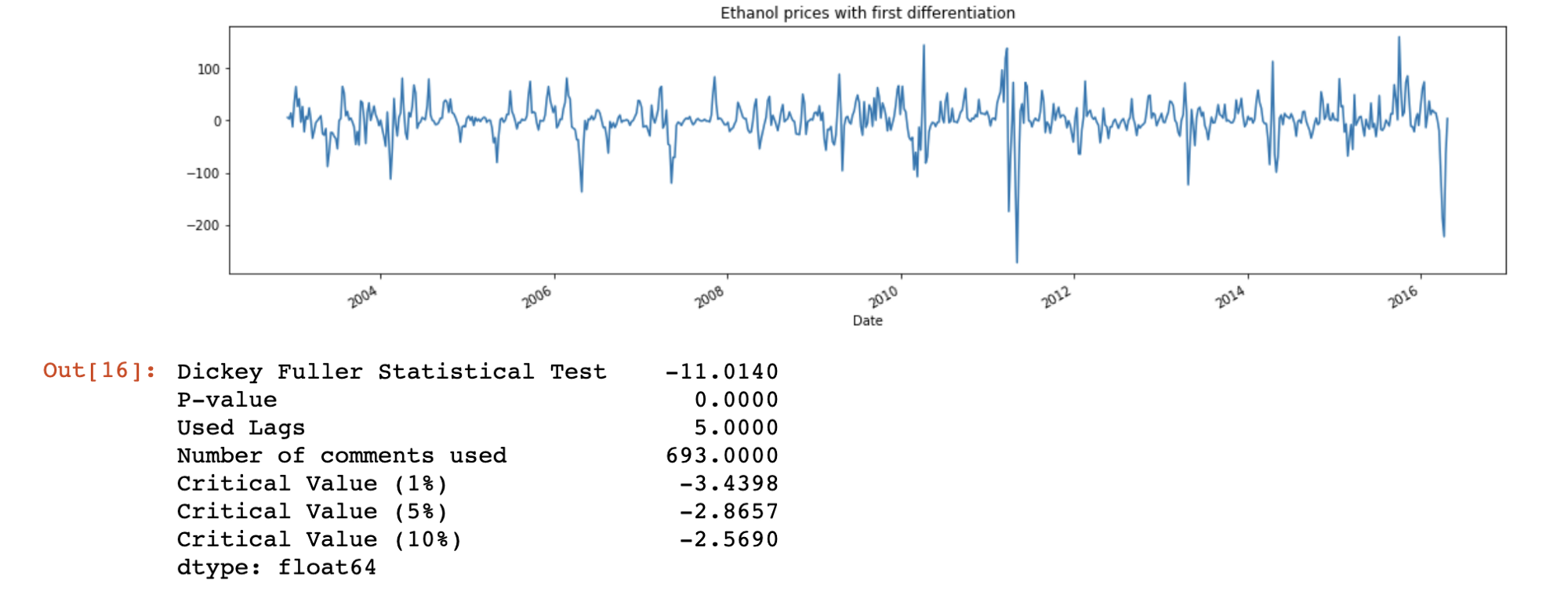### 膨胀率调整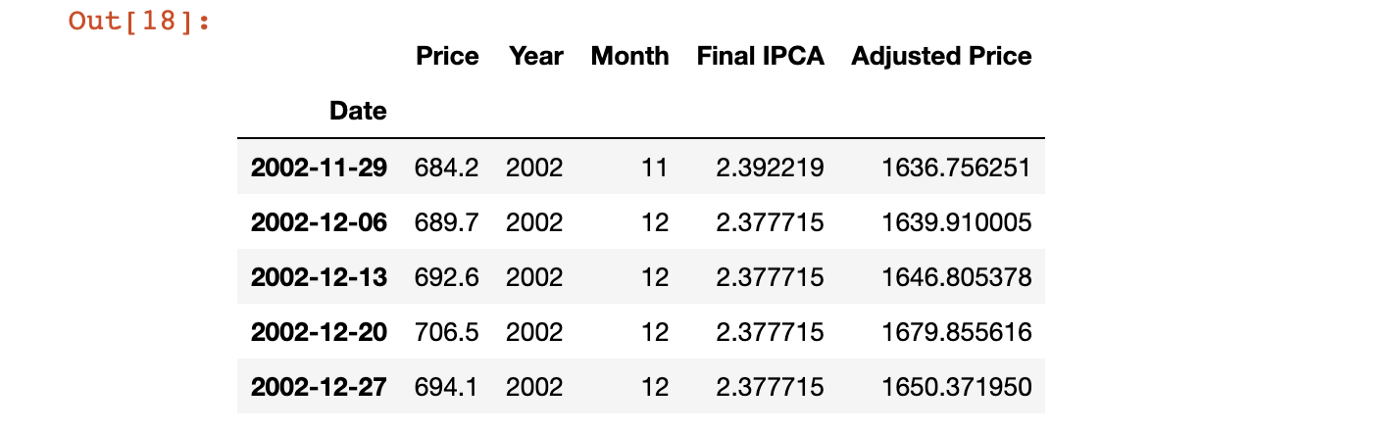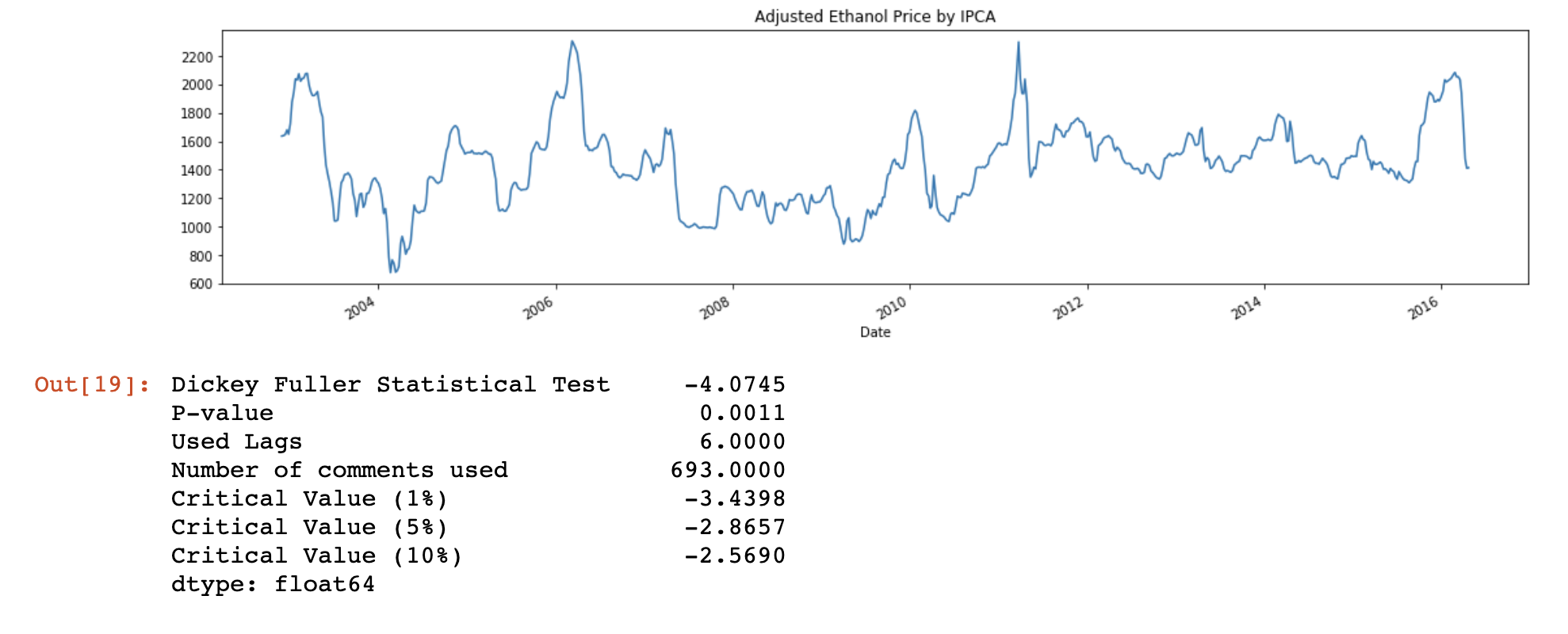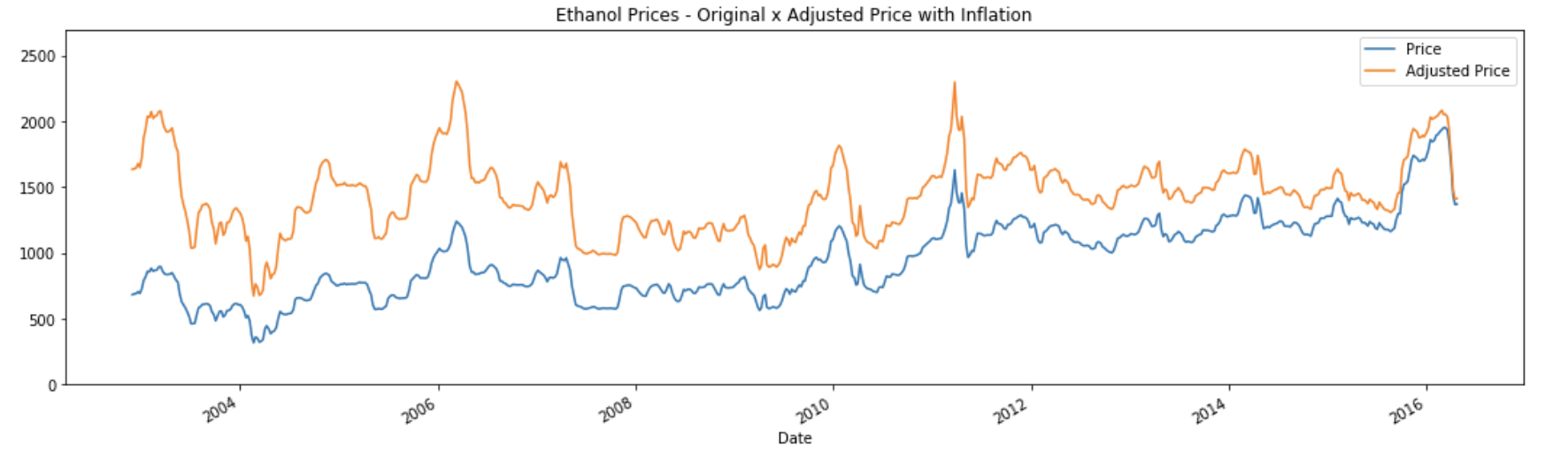## 减小方差

### 对数变换

price1 = np.log(52.5) - np.log(50)
price2 = np.log(105) - np.log(100)
printf('The percentage variation of the first example is {round(price1*100,1)} and the second is {round(price2*100,1)}')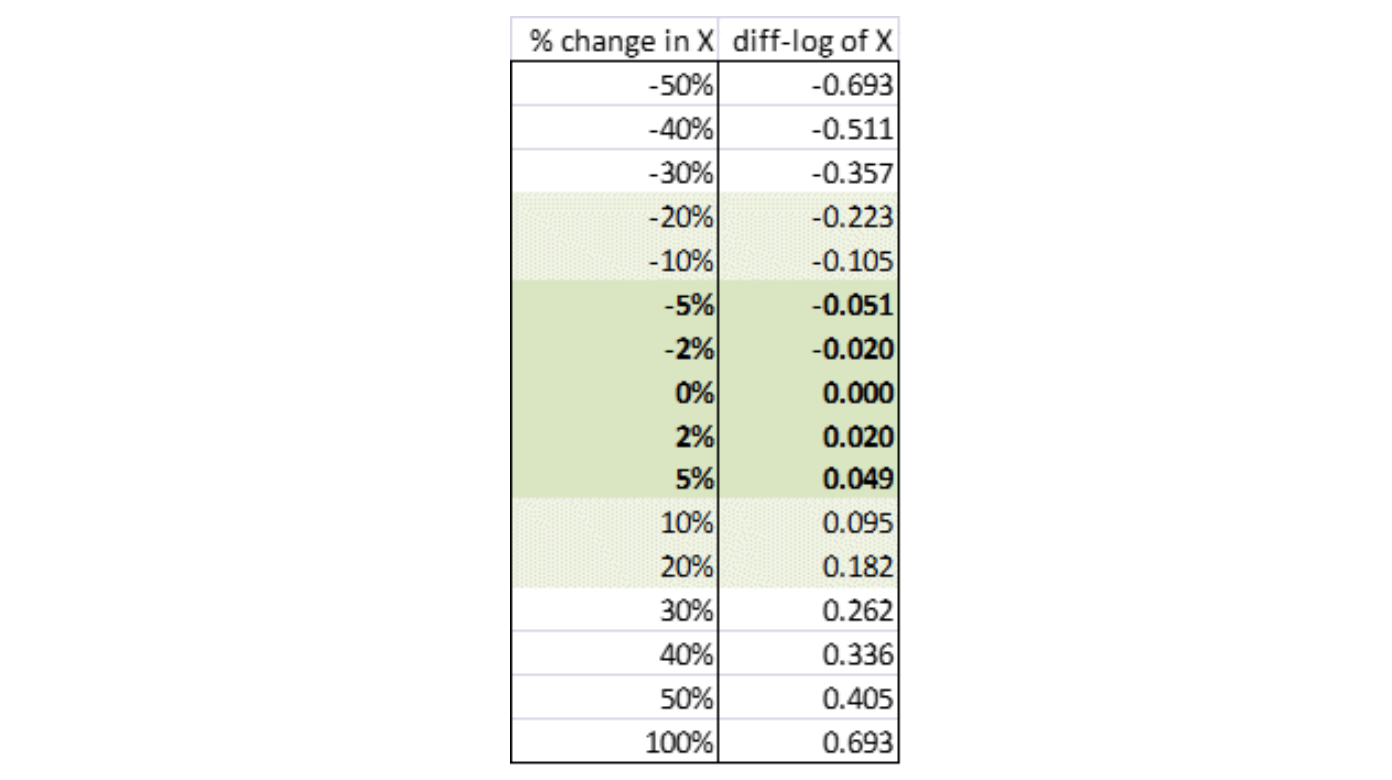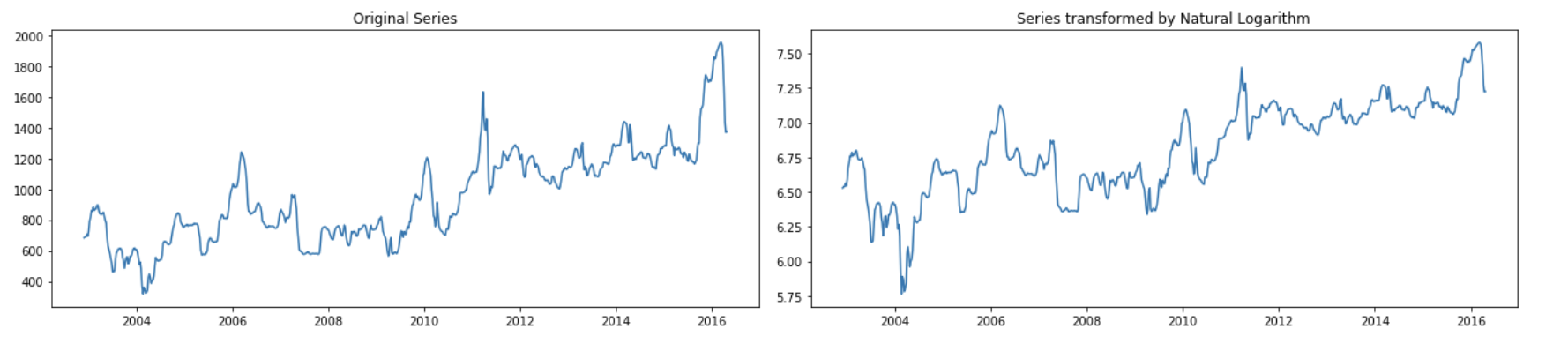### Box-Cox 变换（指数变换）

Box-Cox 转换也是一种转换序列的方法，lambda（λ）的值是用于转换序列的参数。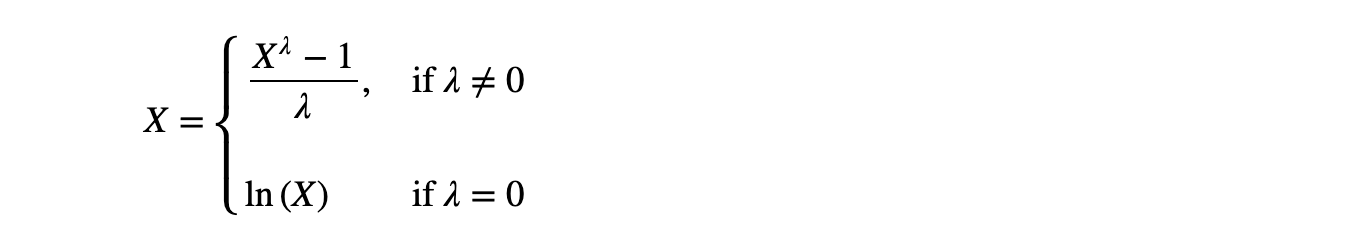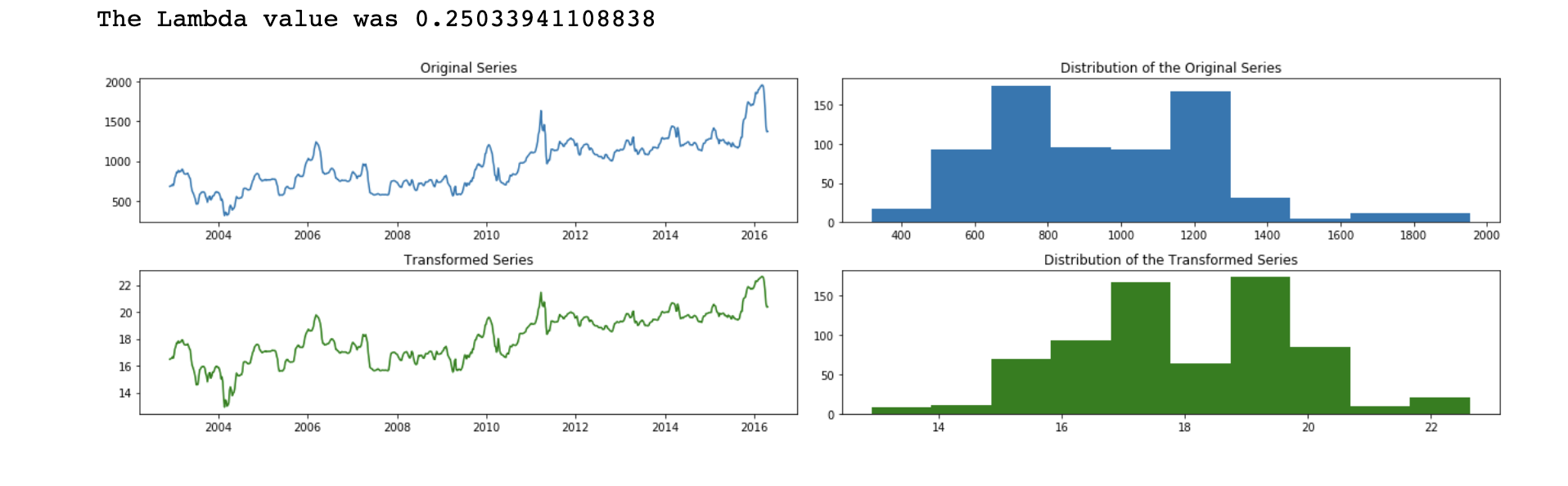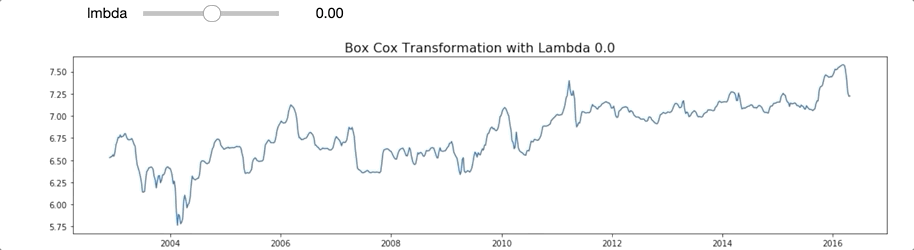### 寻找相关时滞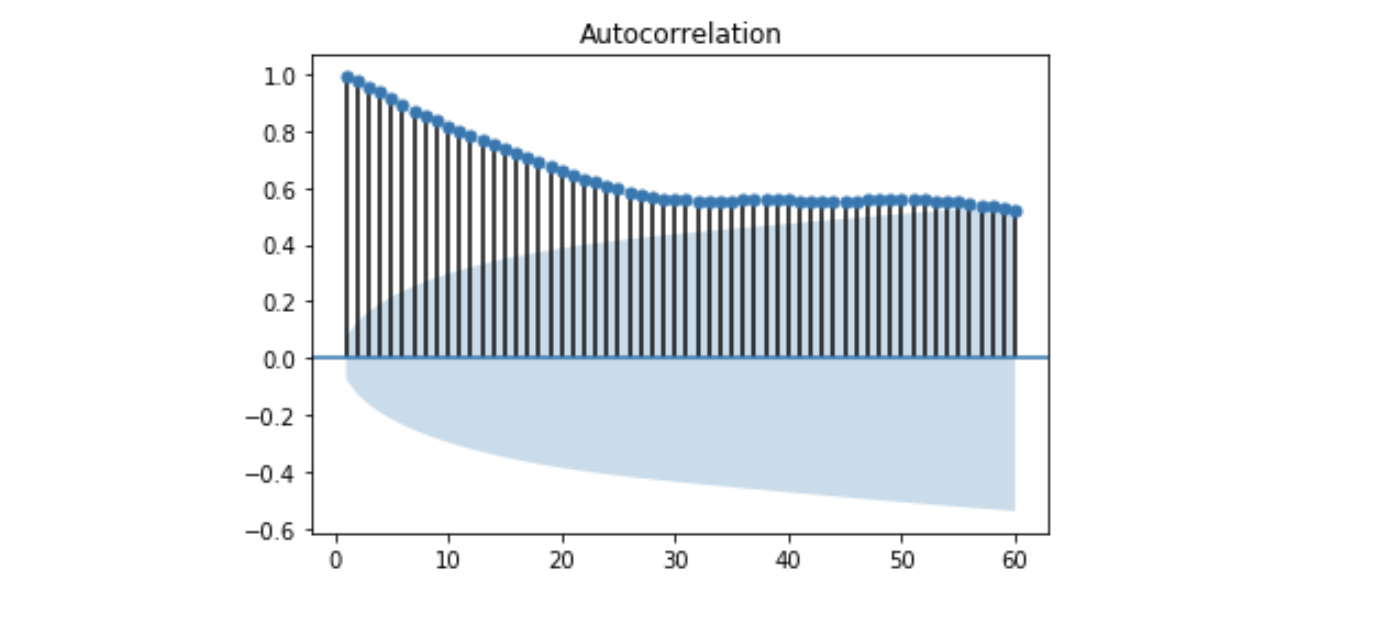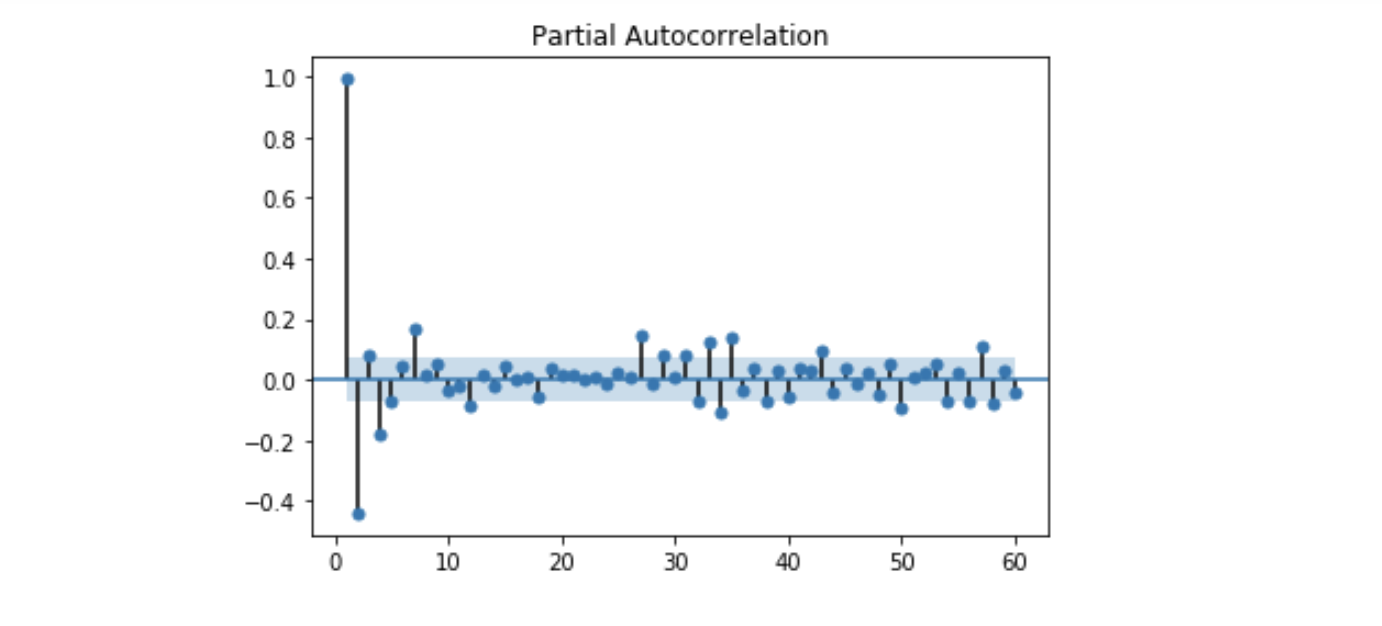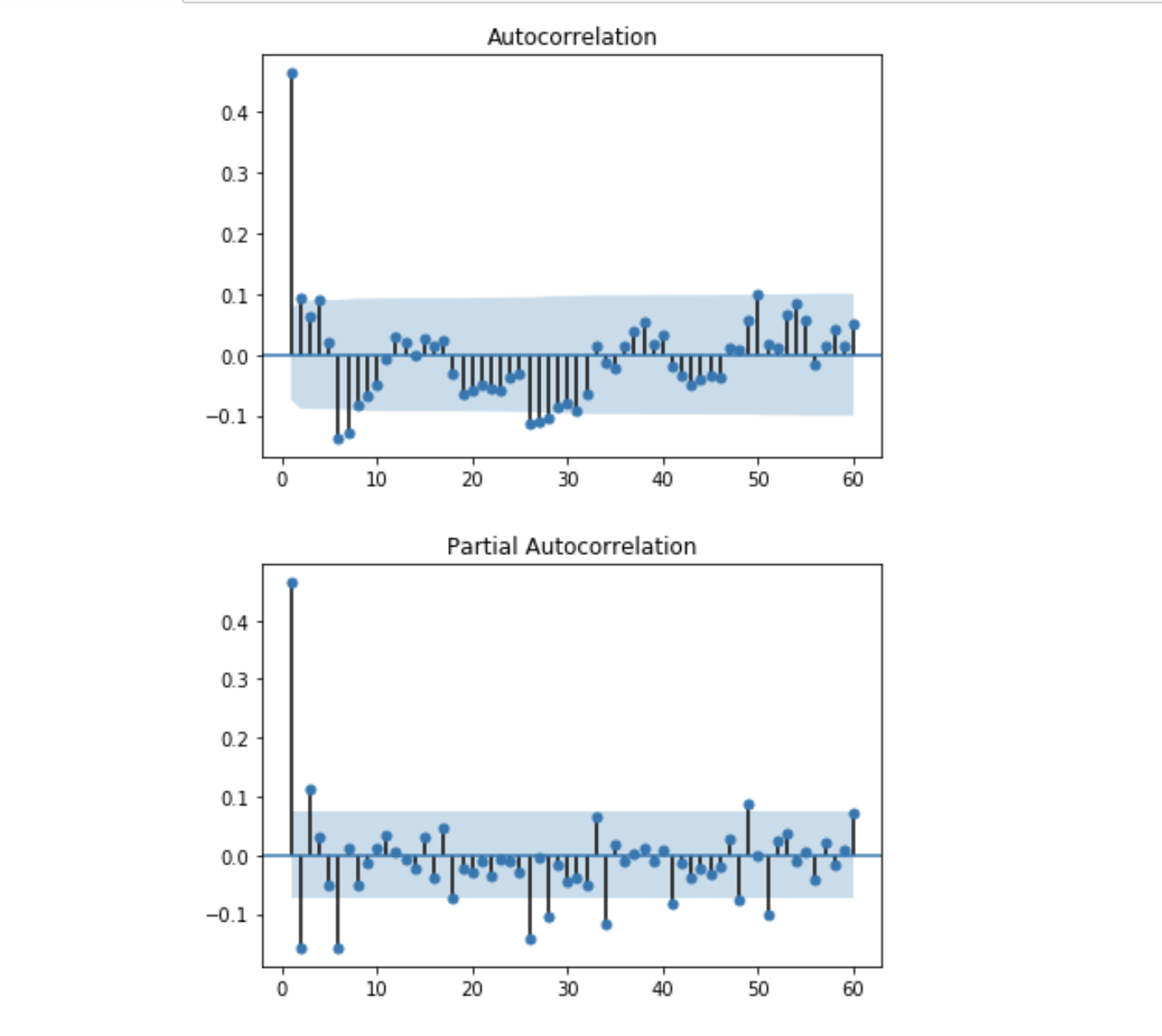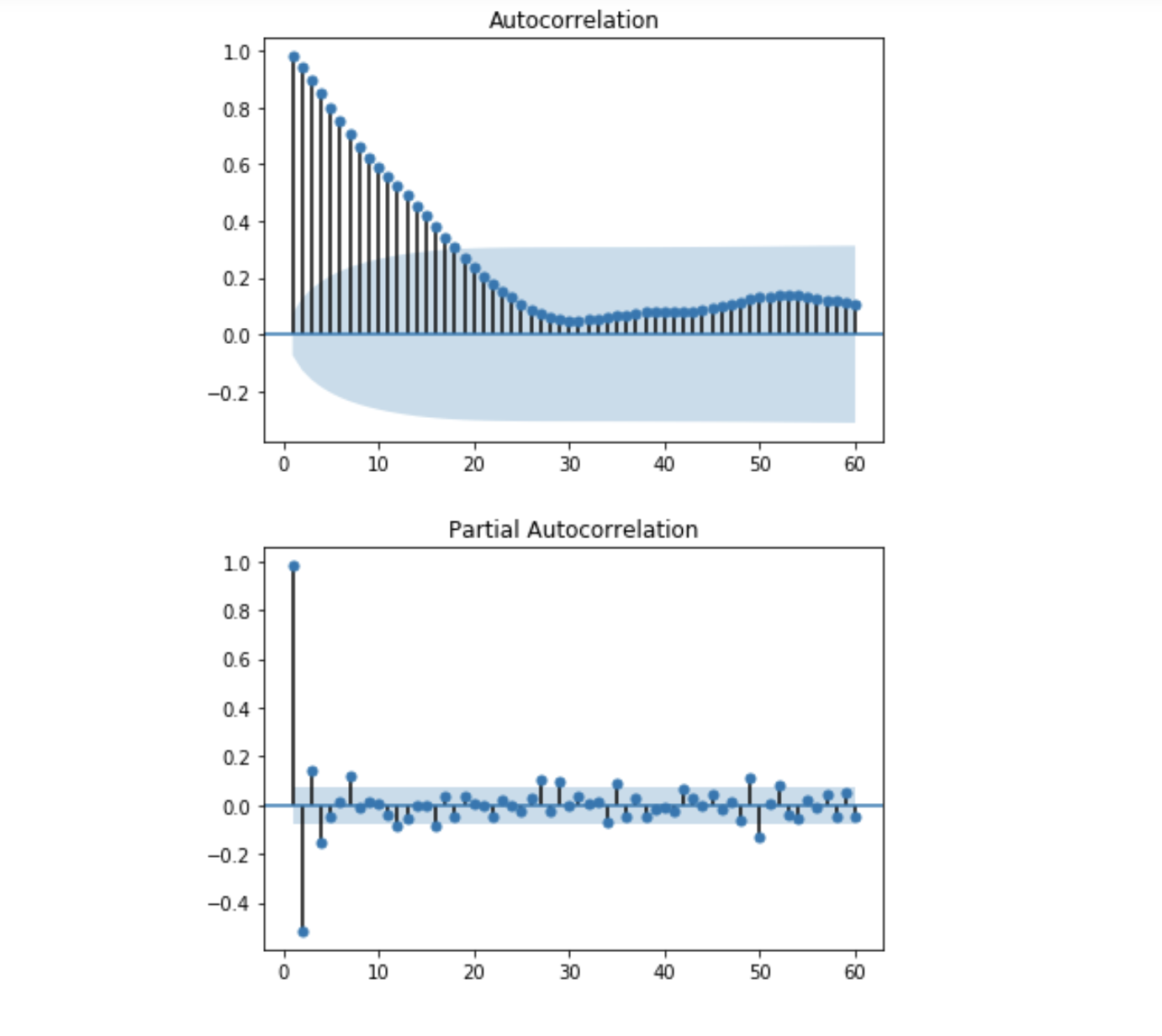## 模型评价指标

### MAE——平均绝对误差

a = np.array([1,2,3,4,5])
b = np.array([5,4,3,2,1])

error = a - b

MFE = error.mean()
MAE = np.abs(error).mean()

print(f'The error of each model value looks like this: {error}')
print(f'The MFE error was {MFE}, the MAE error was {MAE}')


### MAPE——平均绝对百分误差#Libraries to create the function:
from math import sqrt
from sklearn.metrics import mean_squared_error,mean_absolute_error, mean_absolute_error

def check_error(orig, pred, name_col='', index_name=''):
bias = np.mean(orig - pred)
mse = mean_squared_error(orig, pred)
rmse = sqrt(mean_squared_error(orig, pred))
mae = mean_absolute_error(orig, pred)
mape = np.mean(np.abs((orig - pred) / orig)) * 100
error_group = [bias, mse, rmse, mae, mape]
serie = pd.DataFrame(error_group, index=['BIAS','MSE','RMSE','MAE', 'MAPE'], columns=[name_col])
serie.index.name = index_name
return serie


https://en.wikipedia.org/wiki/Q–Q_plot

def plot_error(data, figsize=(18,8)):

# Creating the column error
data['Error'] = data.iloc[:,0] -data.iloc[:,1]

plt.figure(figsize=figsize)
ax1 = plt.subplot2grid((2,2), (0,0))
ax2 = plt.subplot2grid((2,2), (0,1))
ax3 = plt.subplot2grid((2,2), (1,0))
ax4 = plt.subplot2grid((2,2), (1,1))

#Plotting actual and predicted values
ax1.plot(data.iloc[:,0:2])
ax1.legend(['Real','Pred'])
ax1.set_title('Real Value vs Prediction')

# Error vs Predicted value
ax2.scatter(data.iloc[:,1], data.iloc[:,2])
ax2.set_xlabel('Predicted Values')
ax2.set_ylabel('Residual')
ax2.set_title('Residual vs Predicted Values')

## Residual QQ Plot
sm.graphics.qqplot(data.iloc[:,2], line='r', ax=ax3)

# Autocorrelation Plot of residual
plot_acf(data.iloc[:,2], lags=60, zero=False, ax=ax4)
plt.tight_layout()
plt.show()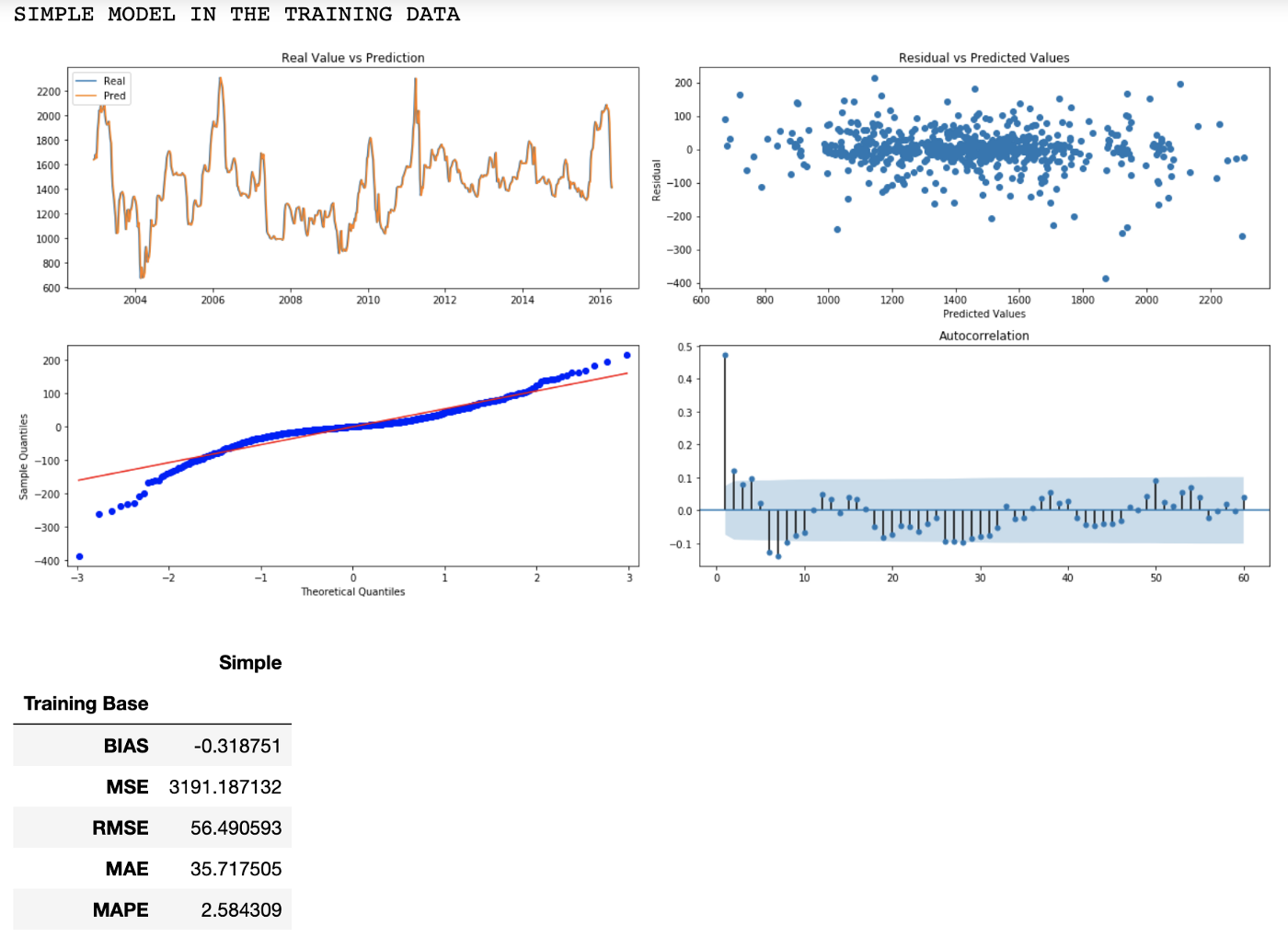QQ 图显示了有一些比理论上要大些（包括正负值）的残差，这些是所谓的异常值，但在第一，第六和第七个滞后中仍然存在明显的自相关，这可以用于改进模型。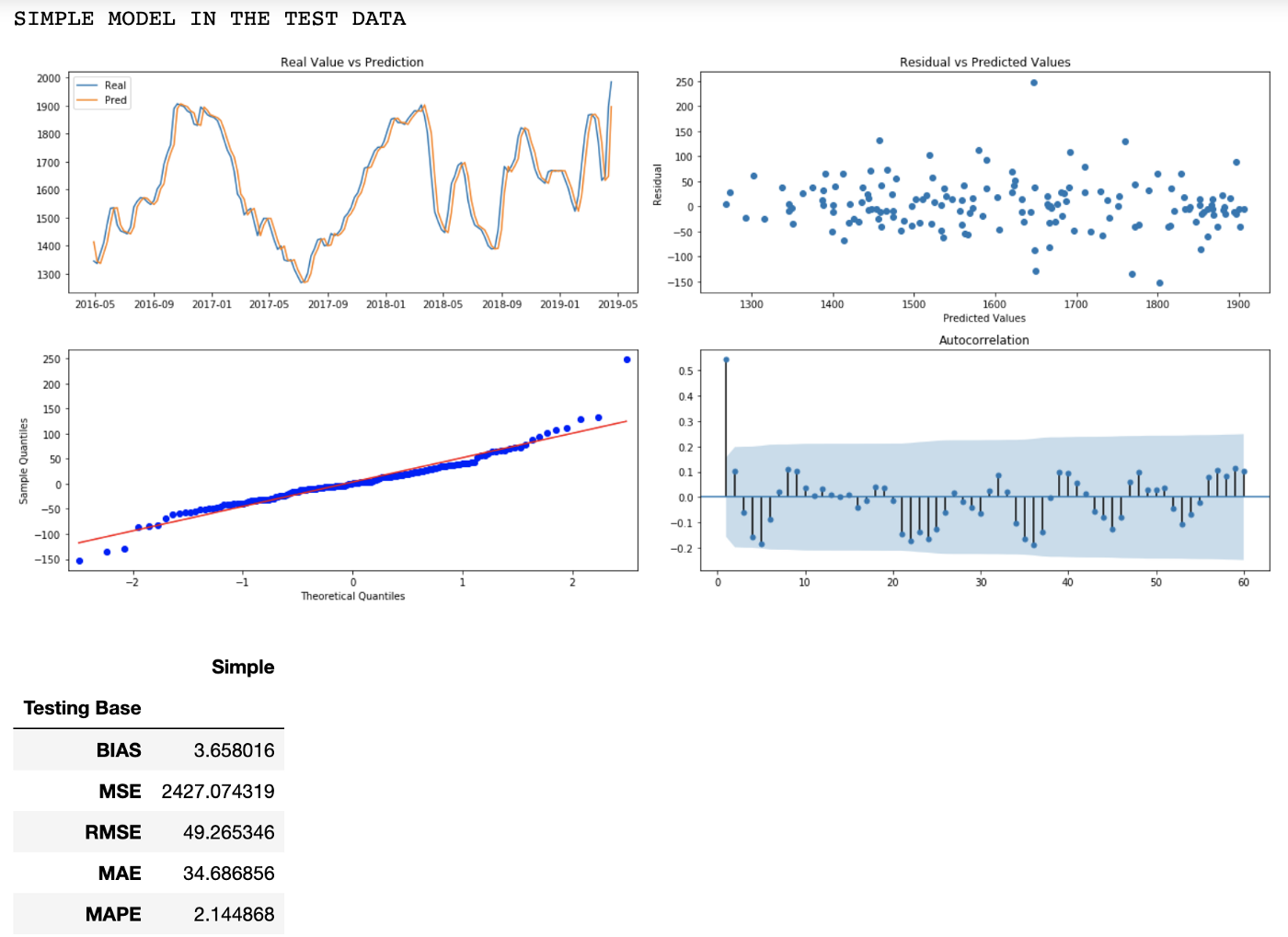RMSE 和 MAE 的误差与训练数据相似，QQ 图与残差更符合理论值，可能是由于与训练数据相比样本值较少。

### 简单平均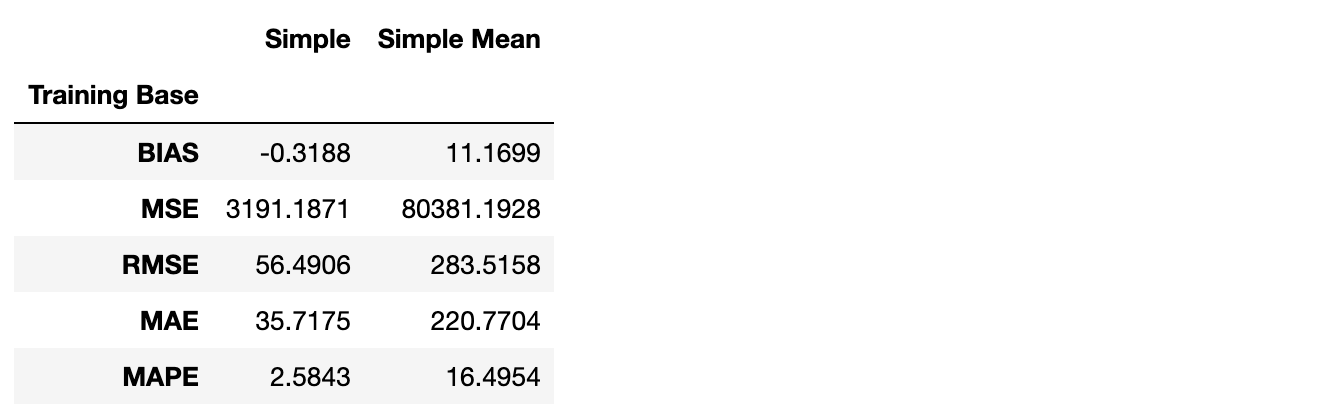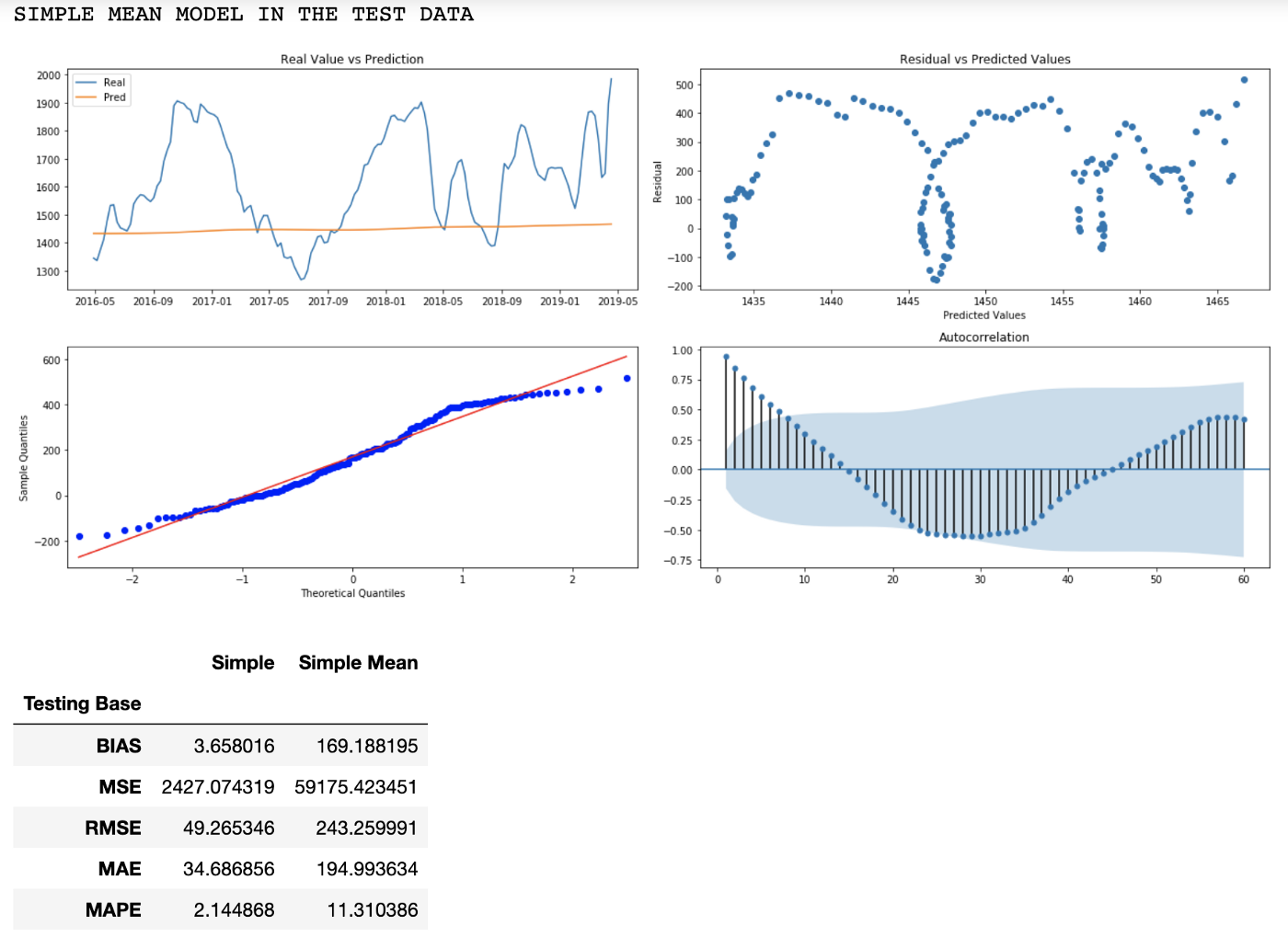### 简单滑动平均：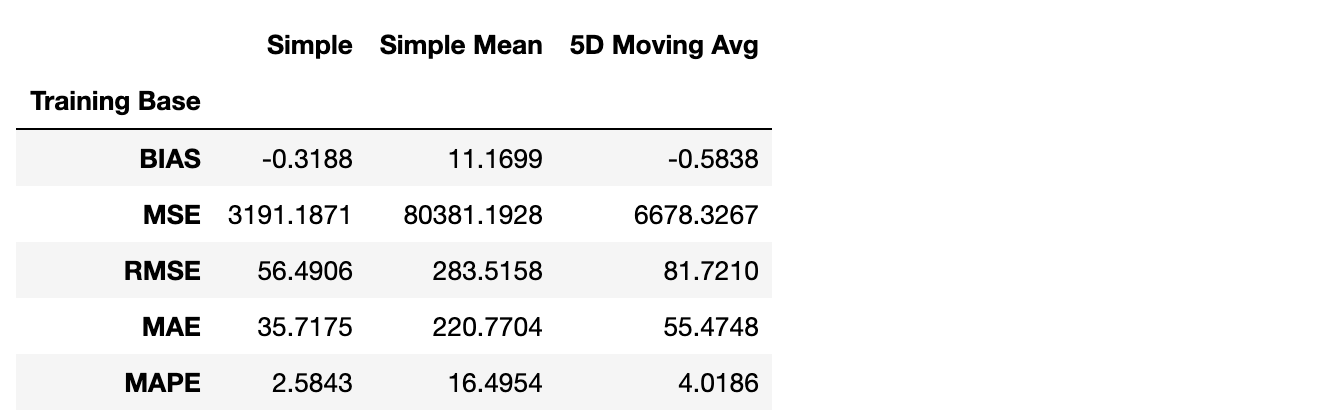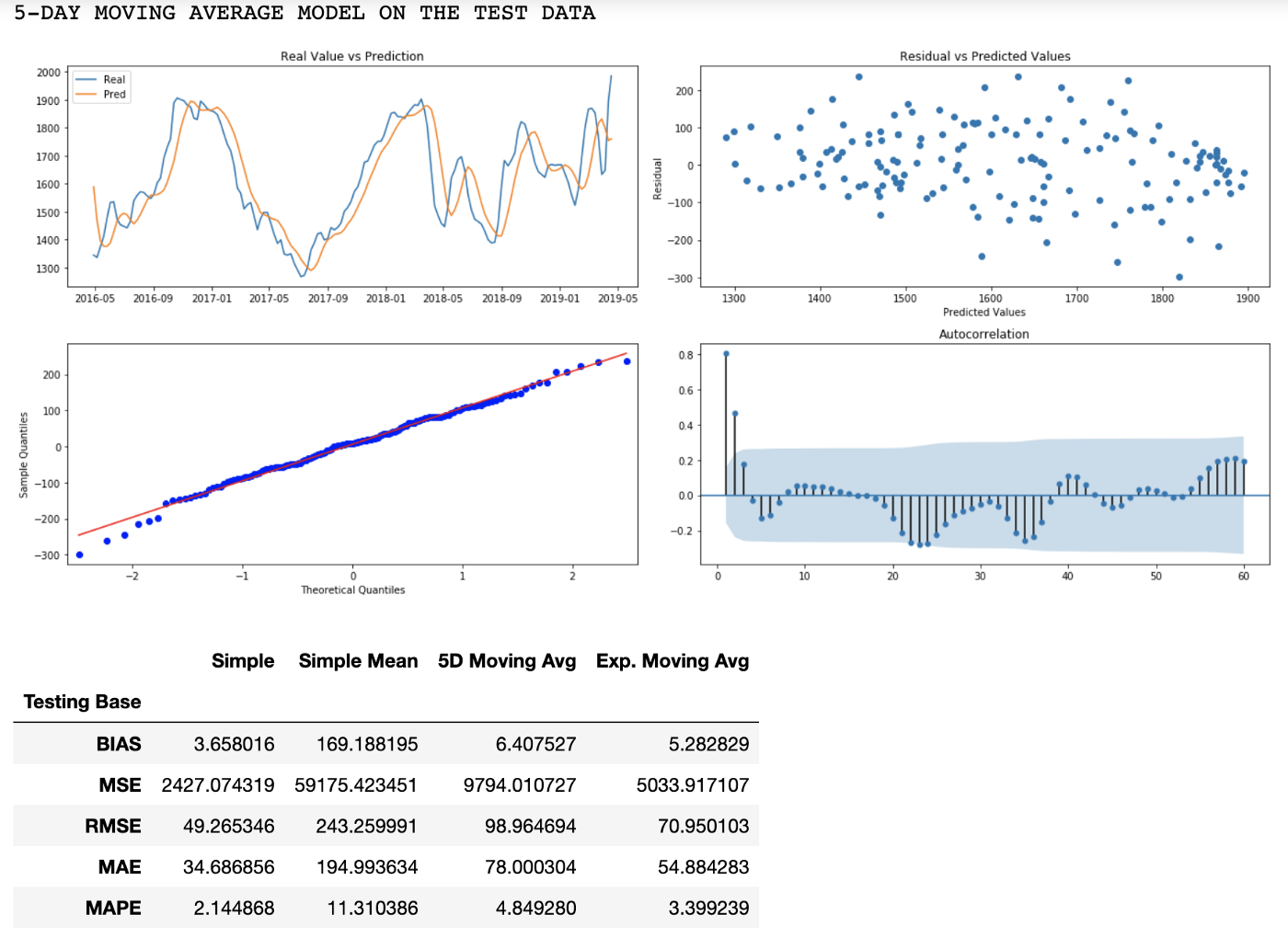### 指数滑动平均: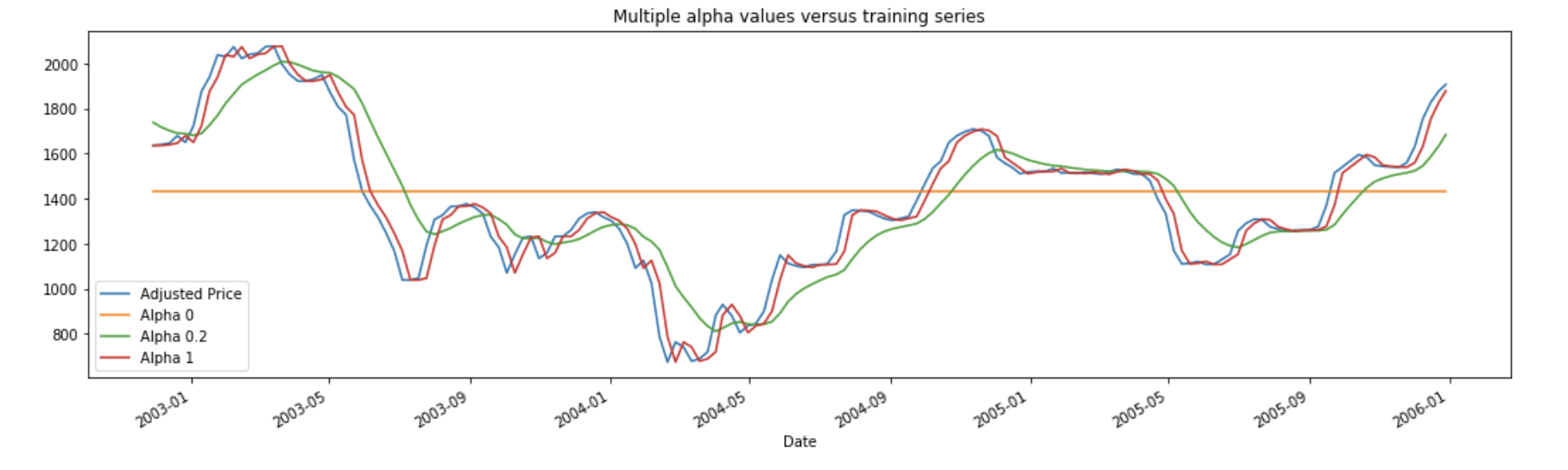EMM 预测中的平均数据周期为 1 /α。 例如，当 α= 0.5 时，滞后相当于 2 个周期; 当 α= 0.2 时，滞后是 5 个周期; 当 α= 0.1 时，滞后是 10 个周期，依此类推。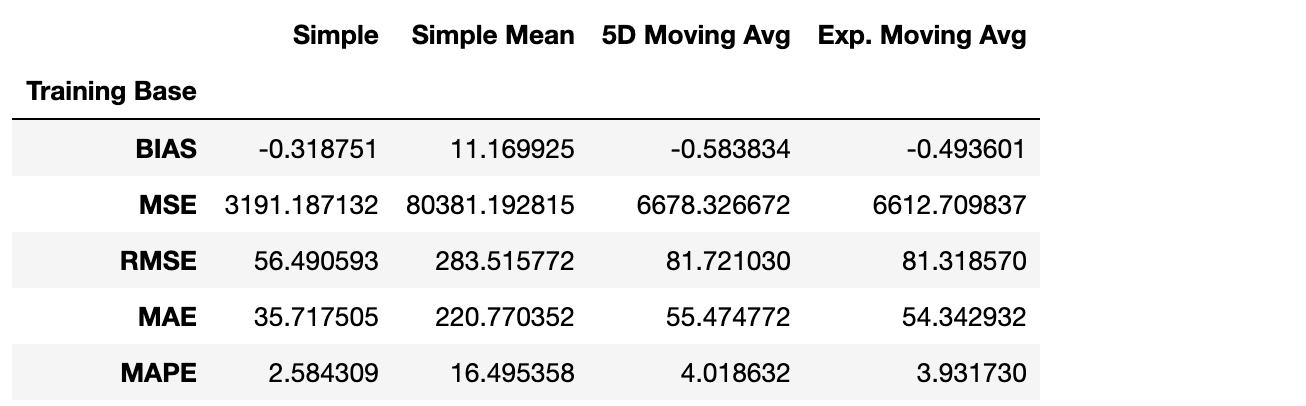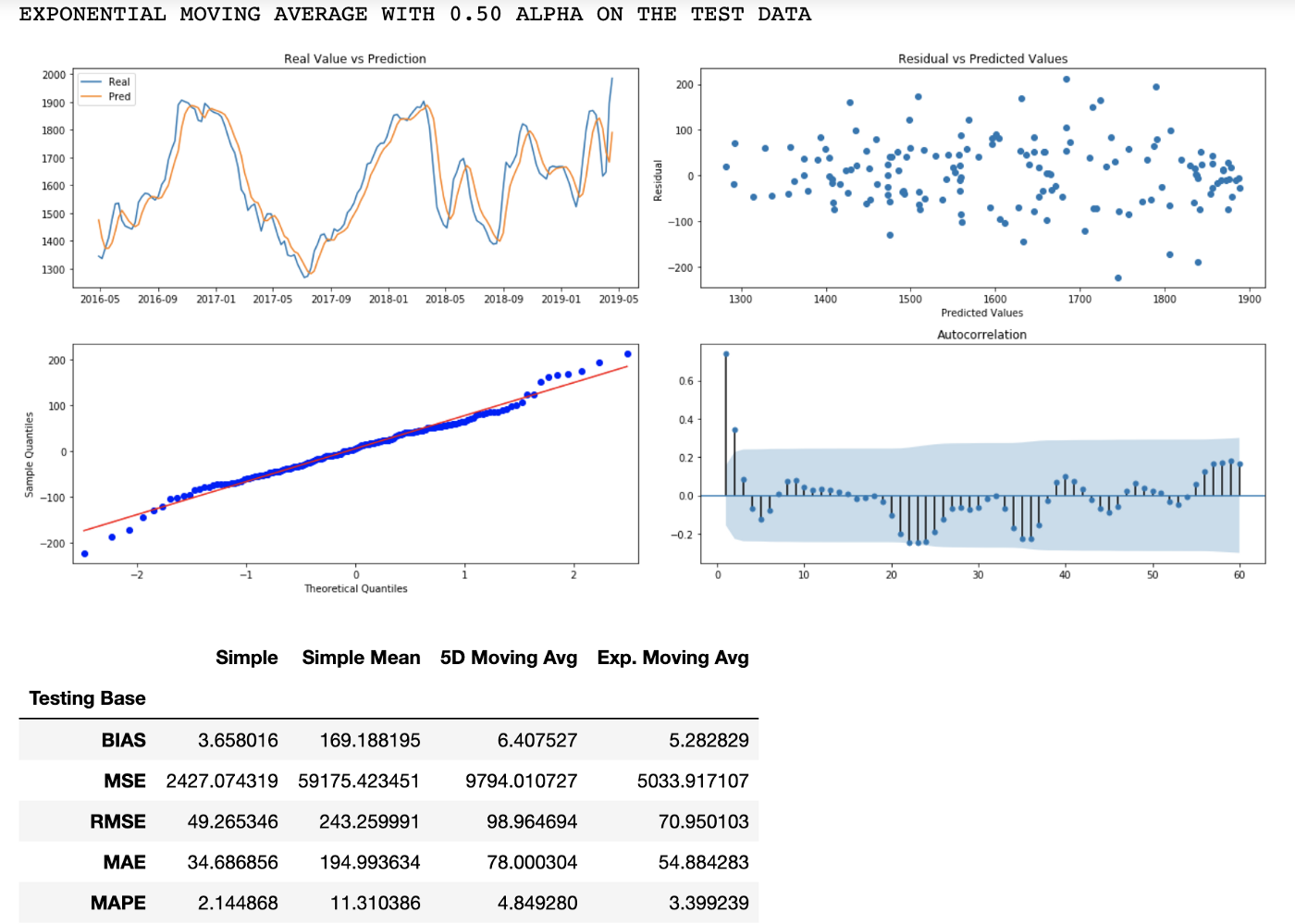### 自回归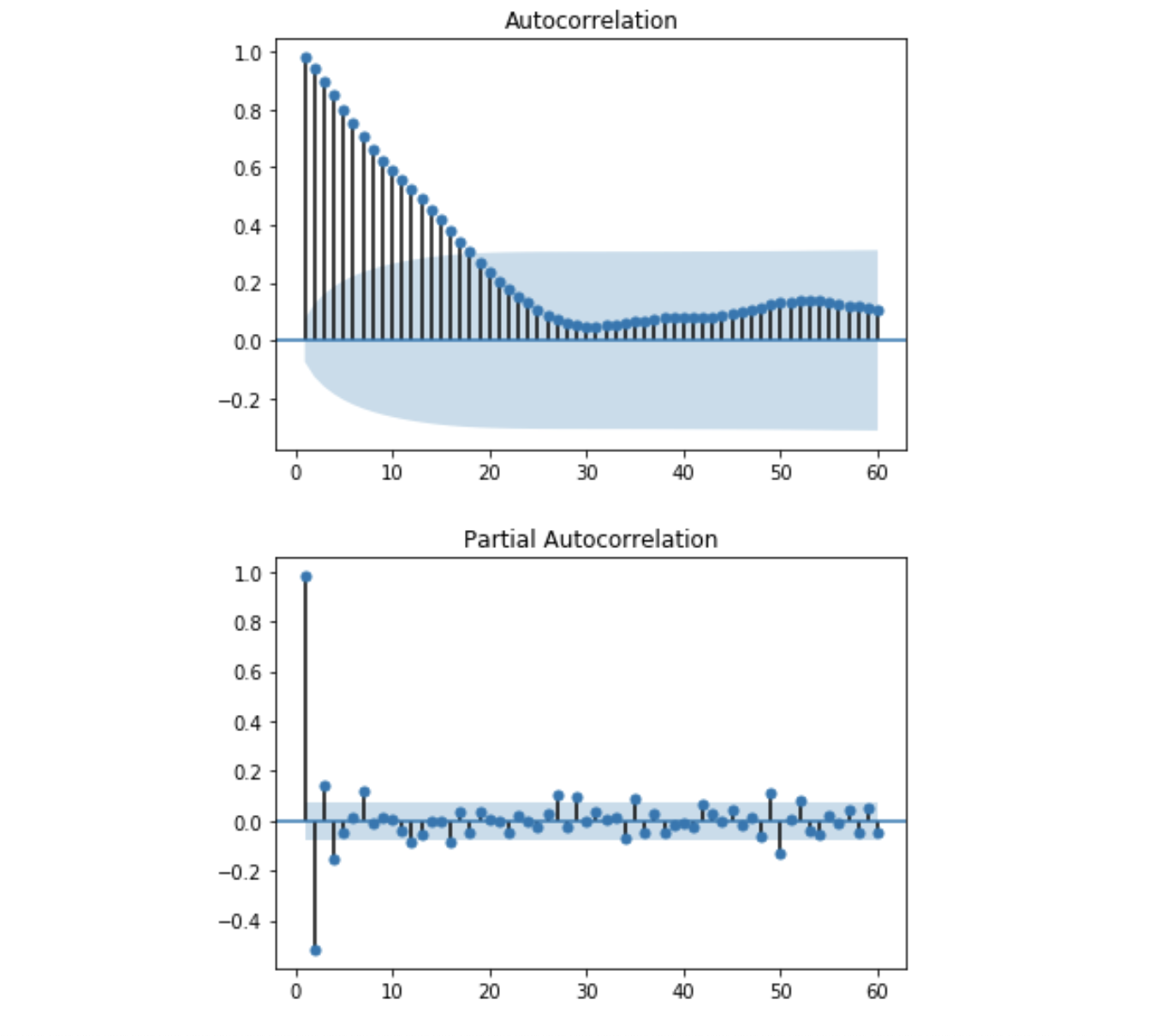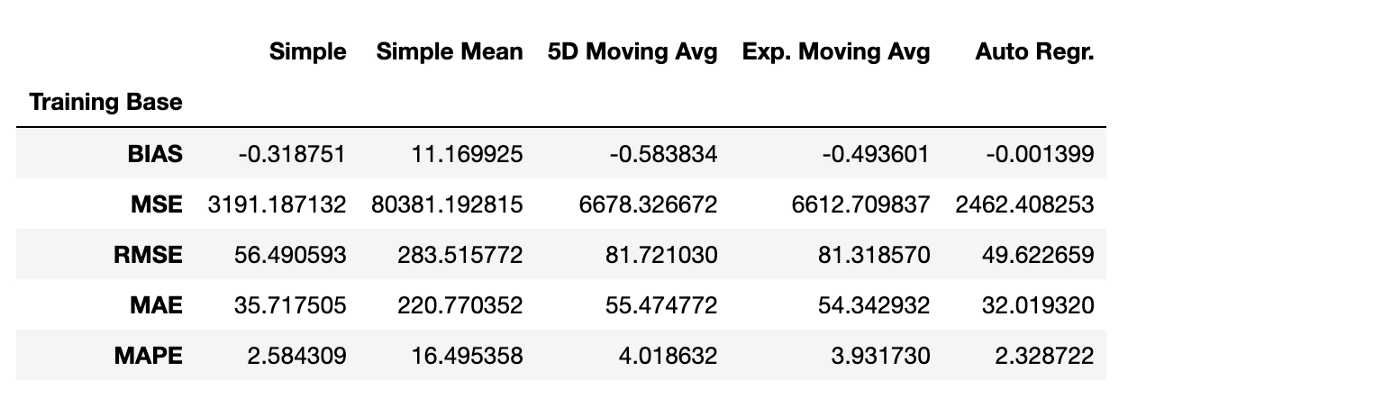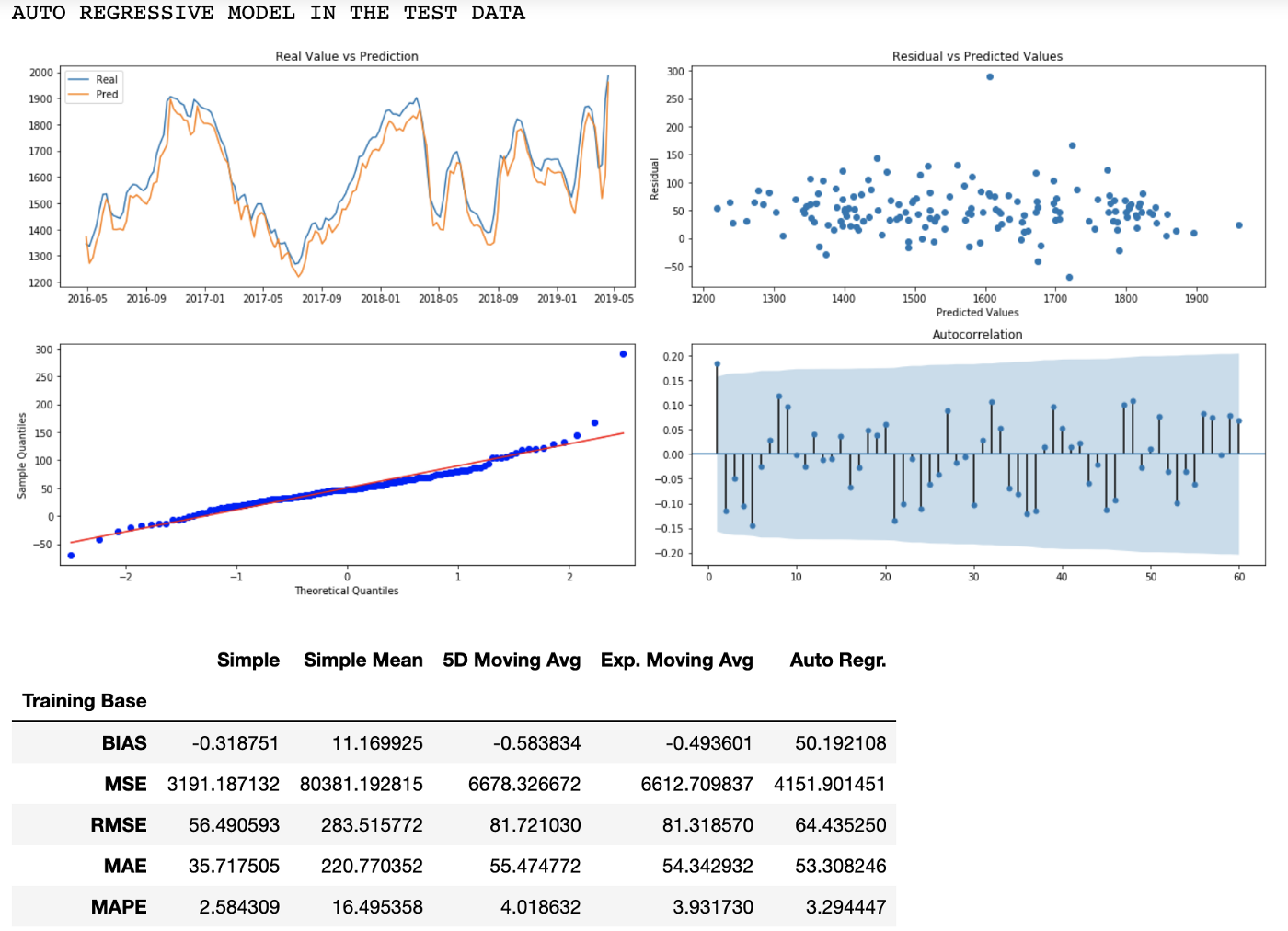## 结束语

https://www.kaggle.com/leandrovrabelo/climate-change-forecast-sarima-model